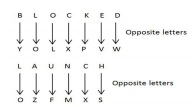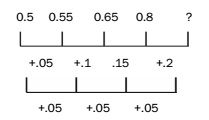# RRB ALP 2018 Practice Test Papers | Reasoning Questions (Day-20)

Dear Aspirants, Here we have given the Important RRB ALP & Technicians Exam 2018 Practice Test Papers. Candidates those who are preparing for RRB ALP 2018 can practice these Reasoning Questions to get more confidence to Crack RRB 2018 Examination.

[WpProQuiz 1758]

### Click “Start Quiz” to attend these Questions and view Solutions

1. Which of the following is different from the others?
1. CJL
2. SZB
3. AHJ
4. XEH
1. Three of the following four options are alike in a certain way and thus form a group. Which is the one that does not belong to that group?
1. Triumph
2. Win
3. Success
4. Growth
1. Three of the following four options are alike in a certain way and so form a group. Which is the one that does not belong to that group?
1. Fast
2. Quick
3. Sudden
4. Rapid
1. Select the set of letters from the given alternatives which, when sequentially placed at the gaps in the given letter series, completes it. kl_ml_klm_llklmmll_lmmllklmm_l
1. mlmlk
2. mlmll
3. mlmkl
4. lmmll
1. If TEMPER is coded as 315718 and RASTER is coded as 890318, what will be the code for ATTEMPT?
1. 9331583
2. 9331573
3. 9331577
4. 9335173
1. X is the father of Y and Z. Y is the son of X, but Z is not the son of X. How is Z related to Y?
1. Niece
2. Sister
3. Daughter
1. If ‘+’ is ‘÷’, ‘–’ is ‘×’, ‘×’ is ‘+’ and ‘÷’ is ‘–’, then what will be the value of 36 + 12 – 6 × 2 ÷ 10?
1. 100
2. 50
3. 10
4. 30
1. BLOCKED: YOLXPVW::?: OZFMXS
1. DEBATE
2. RESULT
3. LABOUR
4. LAUNCH
1. 0.5, 0.55, 0.65, 0.8,?
1. 0.9
2. 0.82
3. 1
4. 0.95
1. How many words with or without meaning can be formed by using all the letters of the word ‘REFUND’, if repetition of letters is not allowed?
1. 960
2. 804
3. 750
4. 720

By following the English alphabet, we can see that the position of the second letter is 7 ahead of the first letter and the position of the third letter is 2 ahead of the second letter. All options except XEH follow this rule.

Except ‘growth’ all others (‘triumph’, ‘win’, and ‘success’) are synonyms.

Except ‘sudden’, all others are synonyms and represent degrees of speed.

The given series is formed by repeating the set ‘klmmll’. So, option 3 is correct.

According to the given codes, code for A is 9, T is 3, E is 1, M is 5 and P is 7. Thus, the code for ATTEMPT is 9331573.

From the above statements, it is clear that Y and Z are the children of X. It is also mentioned that Y is a son and Z is not the son of X. Therefore, it can be said that Z is the daughter of X and the sister of Y.

After replacing with correct symbols, 36 + 12 – 6 × 2 ÷ 10 will be written as 36 ÷ 12 × 6 + 2 – 10.

Now, 36 ÷ 12 × 6 + 2 – 10 = 3 × 6 + 2 – 10 = 20 – 10 = 10The number in place of? should be: 0.8 + 0.2 = 1.

There are 6 distinct letters in the word ‘REFUND’  Number of possible words = 6! = 720.

RRB ALP 2018 Practice Test Papers | Reasoning Questions (Day-1)

RRB ALP 2018 Practice Test Papers | Reasoning Questions (Day-2)

RRB ALP 2018 Practice Test Papers | Reasoning Questions (Day-3)

RRB ALP 2018 Practice Test Papers | Reasoning Questions (Day-4)

RRB ALP 2018 Practice Test Papers | Reasoning Questions (Day-5)

RRB ALP 2018 Practice Test Papers | Reasoning Questions (Day-6)

RRB ALP 2018 Practice Test Papers | Reasoning Questions (Day-7)

RRB ALP 2018 Practice Test Papers | Reasoning Questions (Day-8)

RRB ALP 2018 Practice Test Papers | Reasoning Questions (Day-9)

RRB ALP 2018 Practice Test Papers | Reasoning Questions (Day-10)

RRB ALP 2018 Practice Test Papers | Reasoning Questions (Day-11)

RRB ALP 2018 Practice Test Papers | Reasoning Questions (Day-12)

RRB ALP 2018 Practice Test Papers | Reasoning Questions (Day-13)

RRB ALP 2018 Practice Test Papers | Reasoning Questions (Day-14)

RRB ALP 2018 Practice Test Papers | Reasoning Questions (Day-15)

RRB ALP 2018 Practice Test Papers | Reasoning Questions (Day-16)

RRB ALP 2018 Practice Test Papers | Reasoning Questions (Day-17)

RRB ALP 2018 Practice Test Papers | Reasoning Questions (Day-18)

RRB ALP 2018 Practice Test Papers | Reasoning Questions (Day-19)